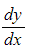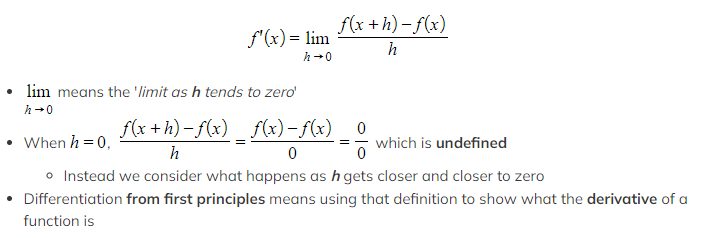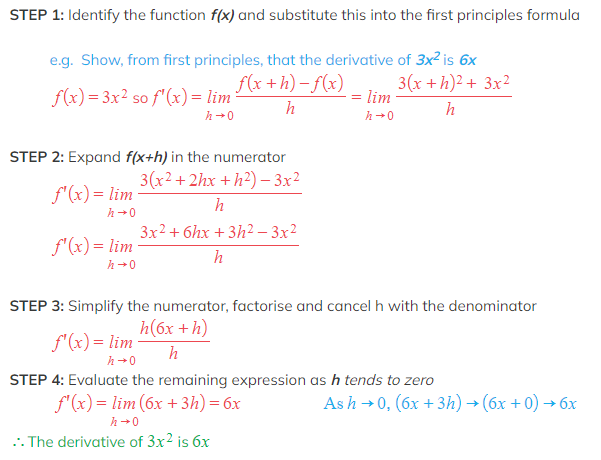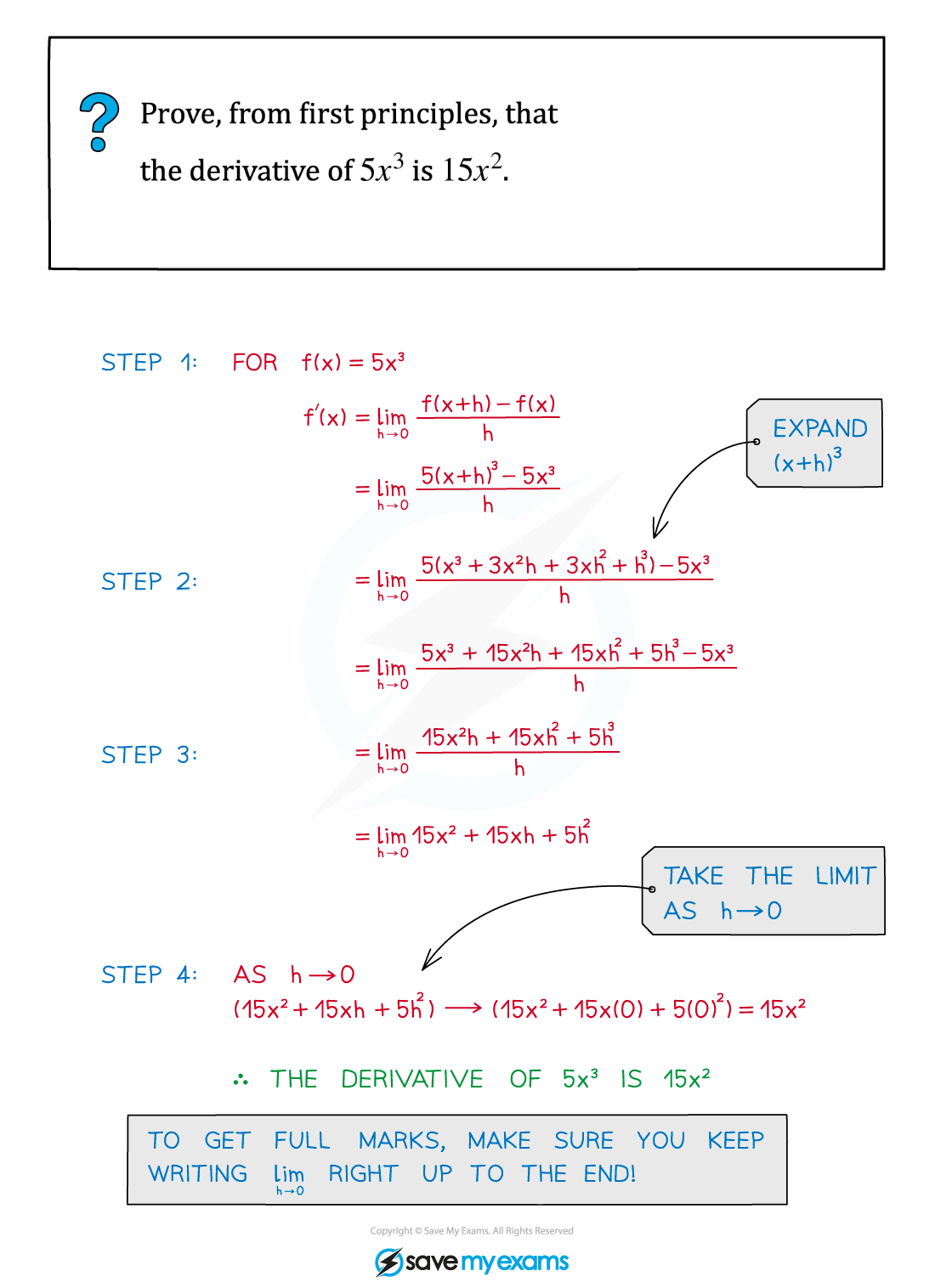# AQA A Level Maths: Pure复习笔记7.1.2 First Principles Differentiation

### First Principles Differentiation

#### What is the derivative or gradient function?

• For a curve y = f(x) there is an associated function called the derivative or gradient function
• The derivative of f(x) is written as f'(x) or• The derivative is a formula that can be used to find the gradient of y = f(x) at any point, by substituting the x coordinate of the point into the formula
• The process of finding the derivative of a function is called differentiation
• We differentiate a function to find its derivative

#### What is differentiation from first principles?

• Differentiation from first principles uses the definition of the derivative of a function f(x)
• The definition is###Exam Tip

• Most of the time you will not use first principles to find the derivative of a function (there are much quicker ways!). However, you can be asked on the exam to demonstrate differentiation from first principles.
• Make sure you can use first principles differentiation to find the derivatives of kx, kx2 and kx3 (where k is a constant).

#### Worked Example